### Probability Class 9th Mathematics AP Board Solution

##### Question 1.A die has six faces numbered from 1 to 6. It is rolled and number on the top face is noted. When this is treated as a random trial.a) What are the possible outcomes?b) Are they equally likely? Why?c) Find the probability of a composite number turning up on the top face.Answer:Here the sample space consist of outcomes = 1,2,3,4,5 and 6Number of possible outcomes = 6a) As sample space consist of all possible outcomes,S (possible outcomes ) = 1,2,3,4,5 and 6b) Yes, since every number (face) has equal possibility of coming up in a random trial they are equally likely. The possibility of each number is.c) let E be the event of composite number turning upthe possible outcomes are 4 and 6number of possible outcomes = 2probability of a composite number turning up is =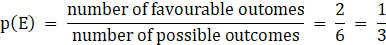Question 2.A coin is tossed 100 times and the following outcomes are recordedHead:45 timesTails:55 times from the experimenta) Compute the probability of each outcomes.b) Find the sum of probabilities of all outcomes.Answer:Given that the coin is tossed 100 times, so sample space consist of 100 possible outcomesHead turns up 45 timesTails turns up 55 timesa) let E be the event of head turning upThen P (E) =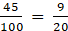Let F be event of tails turning upP (F) =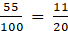b) sum of possibility of outcome=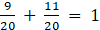Question 3.A spinner has four colours as shown in the figure. When we spin it once, finda) At which colour, is the pointer more likely to stop?b) At which colour, is the pointer less likely to stop?c) At which colours, is the pointer equally likely to stop?d) What is the chance the pointer will stop on white?e) Is there any colour at which the pointer certainly stops?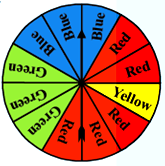Answer:a) The maximum number of partition on the spinner is of colors Red so the pointer is more likely to stop at color RED.b) Color Yellow has only one partition so it is most likely to stop at it the least.c) Since blue and green colors have equal partition on the spinner, it is more likely to stop on these two colors equally likely.d) There is no white color partition on the wheel so there is no chance of spinner stopping on white color.e) No, since it’s a random experiment all the colors have equal chance of being pointed by the spinner.Question 4.A bag contains five green marbles, three blue marbles, two red marbles, and two yellow marbles. One marble is drawn out randomly.a) Are the four different colour outcomes equally likely? Explain.b) Find the probability of drawing each colour marble i.e. , P(green), P(blue), P(red) and P(yellow)c) Find the sum of their probabilities.Answer:Here, sample space consists of 5 green marbles, three blue marbles, two red marbles and two yellow marbles. Total number of marbles are = 5 + 3 + 2 + 2 = 12a) No, the four different color outcomes are not equally likely as green color marbles are maximum in number so the probability of drawing green color marble is most followed by blue color marbles.b) Let G be the event of drawing green marbleThen P (G) =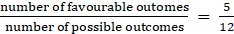Let B be the event of drawing blue marbleThen P (B) =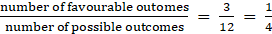Let R be the event of drawing a red marbleThen P (R) =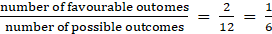Let Y be the event of drawing a yellow marbleThen P(Y) =(c) sum of all of the probabilities is =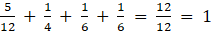Question 5.A letter is chosen from English alphabet. Find the probability of the letters beinga) A vowelb) a letter comes after Pc) A vowel or a consonantd) Not a vowelAnswer:Here sample space consist of 26 alphabets. So total number of possible outcomes = 26Out of these a, e, i , o and u are vowels , ∴ number of vowels are 5And remaining 21 alphabets are consonants.a) let E be the event of drawing a vowelthen, P (E) =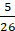b) There are q, r, s ,t , u, v, w , x ,y, z alphabets which comes after letter P.The number of alphabets to come after p = 10And let F be the event of drawing a letter which comes after PP (F) =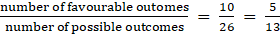(c) Since English alphabets consist of vowels or a consonantThe possibility of drawing a vowel or a consonant will be sum of probability of both the events happening equally likely⇒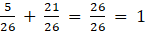Which is also the probability of a sure event.(d) the consonant in English alphabets are b, c, d , f, g, h, , j , k ,l , m ,n, p, q , r ,s ,t ,v, w, x, y, zThe number of consonant are = 21Let E be the event of drawing a consonantThen P ( E) =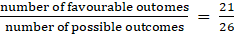Question 6.Eleven bags of wheat flour, each marked 5 kg, actually contained the following weights of flour (in kg):4.97, 5.05, 5.08, 5.03, 5.00, 5.06, 5.08, 4.98, 5.04, 5.07, 5.00Find the probability that any of these bags chosen at random contains more than 5 kg of flour.Answer:Here, the sample space is 11 as the total number of bagsbeing the same.Let E be the event of choosing the bag with weight more than 5 kgThese bags have weight = 5.05, 5.08, 5.03, 5.06, 5.08, 5.04, 5.07Hence Number of favorable outcomes = 7∴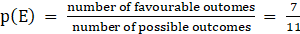Question 7.An insurance company selected 2000 drivers at random (i.e., without any preference of one driver over another) in a particular city to find a relationship between age and accidents. The data obtained is given in the following table: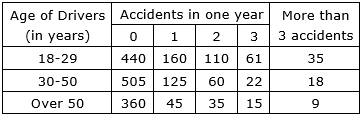Find the probabilities of the following events for a driver chosen at random from the city:i. The driver being in the age group 18-29 years and having exactly 3 accidents in one year.ii. The driver being in the age group of 30-50 years and having one or more accidents in a year.iii. Having no accidents in the year.Answer:here, sample space = 2000 being drivers selected at randomi) Given that the driver belongs to the age group of 18-29 and have exactly 3 accidents in one yearLet E be the event of selecting the driver from this age group with exactly having 3 accidents in a yearSo the possible outcomes will consist 61∴ P ( E) =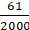ii.) Here the chances of choosing a driver from age group of30 -50 and having 1 or more than 1 accident in a year will be total number of drivers meeting 1 or more than 1 accidents in a year⇒ 125 + 60 + 22 + 18 = 225Let E be the event of choosing the driver from this age group meeting more than 1 accident in a year∴ P (E) =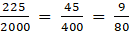iii.) let E be the event of choosing a driver who haven’t meet any accident in a yearThe age group Is not mentioned so we will add all the age group drivers who haven’t meet any accident in the yearThe number of drivers (favorable outcome)= 440 + 505 + 360 = 1305∴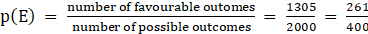Question 8.What is the probability that a randomly thrown dart that hits the square board in shaded region. (side of square = 4cm)(Take π = 22/7 and express in percentage)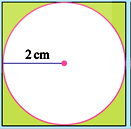Answer:Here, according to the given conditionArea of the square = side2 = 4 × 4 = 16 cm2This is also the possible outcomeArea of the circle = π r2 =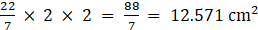Area of the shaded region = area of square – area of circle= 16 – 12.57 = 3.428 cm2which is also a favorable outcomelet E be the event of dart hitting the shaded region∴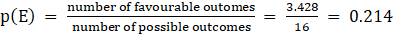= 21.4%

PDF FILE TO YOUR EMAIL IMMEDIATELY PURCHASE NOTES & PAPER SOLUTION. @ Rs. 50/- each (GST extra)

HINDI ENTIRE PAPER SOLUTION

MARATHI PAPER SOLUTION

SSC MATHS I PAPER SOLUTION

SSC MATHS II PAPER SOLUTION

SSC SCIENCE I PAPER SOLUTION

SSC SCIENCE II PAPER SOLUTION

SSC ENGLISH PAPER SOLUTION

SSC & HSC ENGLISH WRITING SKILL

HSC ACCOUNTS NOTES

HSC OCM NOTES

HSC ECONOMICS NOTES

HSC SECRETARIAL PRACTICE NOTES

# 2019 Board Paper Solution

HSC ENGLISH SET A 2019 21st February, 2019

HSC ENGLISH SET B 2019 21st February, 2019

HSC ENGLISH SET C 2019 21st February, 2019

HSC ENGLISH SET D 2019 21st February, 2019

SECRETARIAL PRACTICE (S.P) 2019 25th February, 2019

HSC XII PHYSICS 2019 25th February, 2019

CHEMISTRY XII HSC SOLUTION 27th, February, 2019

OCM PAPER SOLUTION 2019 27th, February, 2019

HSC MATHS PAPER SOLUTION COMMERCE, 2nd March, 2019

HSC MATHS PAPER SOLUTION SCIENCE 2nd, March, 2019

SSC ENGLISH STD 10 5TH MARCH, 2019.

HSC XII ACCOUNTS 2019 6th March, 2019

HSC XII BIOLOGY 2019 6TH March, 2019

HSC XII ECONOMICS 9Th March 2019

SSC Maths I March 2019 Solution 10th Standard11th, March, 2019

SSC MATHS II MARCH 2019 SOLUTION 10TH STD.13th March, 2019

SSC SCIENCE I MARCH 2019 SOLUTION 10TH STD. 15th March, 2019.

SSC SCIENCE II MARCH 2019 SOLUTION 10TH STD. 18th March, 2019.

SSC SOCIAL SCIENCE I MARCH 2019 SOLUTION20th March, 2019

SSC SOCIAL SCIENCE II MARCH 2019 SOLUTION, 22nd March, 2019

XII CBSE - BOARD - MARCH - 2019 ENGLISH - QP + SOLUTIONS, 2nd March, 2019

# HSCMaharashtraBoardPapers2020

(Std 12th English Medium)

HSC ECONOMICS MARCH 2020

HSC OCM MARCH 2020

HSC ACCOUNTS MARCH 2020

HSC S.P. MARCH 2020

HSC ENGLISH MARCH 2020

HSC HINDI MARCH 2020

HSC MARATHI MARCH 2020

HSC MATHS MARCH 2020

# SSCMaharashtraBoardPapers2020

(Std 10th English Medium)

English MARCH 2020

HindI MARCH 2020

Hindi (Composite) MARCH 2020

Marathi MARCH 2020

Mathematics (Paper 1) MARCH 2020

Mathematics (Paper 2) MARCH 2020

Sanskrit MARCH 2020

Sanskrit (Composite) MARCH 2020

Science (Paper 1) MARCH 2020

Science (Paper 2)

Geography Model Set 1 2020-2021

MUST REMEMBER THINGS on the day of Exam

Are you prepared? for English Grammar in Board Exam.

Paper Presentation In Board Exam

How to Score Good Marks in SSC Board Exams

Tips To Score More Than 90% Marks In 12th Board Exam

How to write English exams?

How to prepare for board exam when less time is left

How to memorise what you learn for board exam

No. 1 Simple Hack, you can try out, in preparing for Board Exam

How to Study for CBSE Class 10 Board Exams Subject Wise Tips?

JEE Main 2020 Registration Process – Exam Pattern & Important Dates

NEET UG 2020 Registration Process Exam Pattern & Important Dates

How can One Prepare for two Competitive Exams at the same time?

8 Proven Tips to Handle Anxiety before Exams!